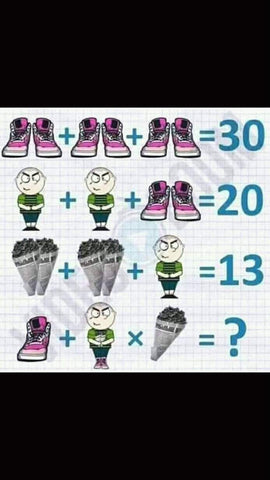# Only A Math Genius can answer this!

Only a Math Genius can answer this 😂We do not own the image. Credits to the owner of the image.

## Here is the answer: 43

You might be wondering why on earth is the answer 43? given that :

Pair of Shoes = 10

Man = 5

2 cones of Icecream = 4

BUT! if you look at the last equation more closely, you'll notice there is something different with the images.

A shoe 5 + (Pair of shoes (worn by the man) 10 + 2 cones of Icecream (held by the man) 4 + Man 5) x A cone of Icecream 2 = 43

5 + (10+4+5)2 =
5 + (19)2 =
5 + 38 =
43

There you have it guys :) We hope you had a fun time trying to solve this riddle.

Have a good one!

Sale

Unavailable

Sold Out